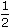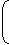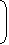# Aptitude - Compound Interest - Discussion

### Discussion :: Compound Interest - General Questions (Q.No.4)

4.

What is the difference between the compound interests on Rs. 5000 for 1years at 4% per annum compounded yearly and half-yearly?

 [A]. Rs. 2.04 [B]. Rs. 3.06 [C]. Rs. 4.80 [D]. Rs. 8.30

Explanation:

C.I. when interest
compounded yearly
 = Rs.5000 x1 + 4x1 +x 4100 100
 = Rs.5000 x 26 x 5125 50
= Rs. 5304.

C.I. when interest is
compounded half-yearly
 = Rs.5000 x1 + 23100
 = Rs.5000 x 51 x 51 x 5150 50 50
= Rs. 5306.04Difference = Rs. (5306.04 - 5304) = Rs. 2.04

 Ravi said: (Aug 13, 2010) Why do we take 2 r in the half-yearly?

 Anjali said: (Oct 14, 2010) Hello, Since Compound Interest (C.I.) = p[1+{(r/2)/(100)}]^2n for half yearly Thus, C.I.= 5000[1+{(4/2)/(100)}]^{2*(3/2)} =5000[1+{2/100}]^(3) =5306.04

 Manisha said: (Nov 12, 2010) How we got 3/2 for half yearly for one and half year. please assist.

 Binnu said: (Jan 11, 2011) According to the question no. of years=1 1/2 so it is equal to 3/2 just(2*1+1)/2

 Sachin said: (Feb 9, 2011) As per formula it should be, C.I. when interest = 5304-5000 = 304 compounded yearly C.I. when interest is = 5306.04-5000 = 306.04 compounded half-yearly Difference = Rs. (306.04 - 304) = Rs. 2.04

 Manikanta said: (Sep 28, 2011) What about the Compound interest per year? Why do we take [ 1/2 * 4 ] ?

 Mehul said: (Dec 31, 2012) Any shortcut method?

 Deekshit said: (Jul 10, 2013) But the given question is difference b/w year and and half-yearly not as one and half-yearly so the answer is 2.04.

 Srimoyee said: (Jul 17, 2013) The formula is slightly incorrect. Amount = P(1+R/100)^1 + [1+(1/2*R)/100] then C.I = AMOUNT - SUM. Same is in the case of half-yearly. Amount = P(1+R/200)^2*3/2. THEN CI = AMOUNT - SUM.

 Ritesh Kasat said: (Jul 29, 2013) p(1+r/100)^n is value of amount and not compound interest. However if we subtract two amounts, since the principal remains same, we will still get the difference between compound interest only.

 Afsha said: (Dec 13, 2013) Finding it tuff to understand can anyone please make it easy for me from first step.

 Tarun said: (Feb 2, 2014) When interest is compounded annually, Amount = p[1+(r/100)]^t. When interest is compounded half yearly, Amount = p[1+(r/2x100)]^2t.

 Qutub said: (Feb 6, 2014) How "C. I. When interest compounded yearly" is calculated?

 Shakti said: (Mar 19, 2014) When interest is compounded annually~ Yearly, Amount(A) = p[1+(r/100)]^t. CI = P-A.x

 Arslan said: (May 14, 2014) As C.I = [1+4/100]^3/2. = [1+.03]^3/2. = 5302.980298. Why?

 Akshay said: (Jun 24, 2014) Why is is 1+[(1/2)*4/100] when it should be 1+(4/100)^1/2 when the interest is compounded annually.

 Roop said: (Jun 27, 2014) Why 1/2*4 instead of (1+4/100)^1/2 ?

 Roop said: (Jun 27, 2014) @Akshay and @Arslan. When time is in fraction that is 3/2 then the formula is: A = P[(1+R/100)*{1+(R/2)/100).

 Sneh said: (Jun 29, 2014) How we can compound half yearly means I did not understand how 3 can come means n=3 ?

 Divya said: (Jul 8, 2014) Compound interest indicates interest on interest. So we can calculate it as: CI1 = 5000*(4/100)*1 = 200. But I don't know to calculate, CI2 for 1/2 year. Such that compound interest CI = CI1+CI2.

 Simran said: (Aug 24, 2014) How to solve for year and a half when asked in a question please tell me now?

 Shivanath said: (Sep 25, 2014) What is the compound interest formula for full year. Tell the general nth year formula?

 Deepthi said: (Nov 4, 2014) How can we multiply 1/2 along with 4/100 when compounded annually?

 Deepthi said: (Nov 4, 2014) What is the actual equation for CI calculated annually?

 Aswathi said: (Dec 10, 2014) Explanation for the amount when compounded yearly is as below: We learn from our formulas that when interest is compounded, annually but time is in fraction then it should be: Amount = P*(1+r/100)^1*(1+(1/2*4)/100). Please check general formula section 5.

 Gurpreet said: (Feb 6, 2015) For yearly A = P[(1+R/100)*{1+(R/2)/100). Then CI =A-P =304. For half-yearly A = P(1+(R/2)/100)^3. Then CI =A-P =306.04. After that difference = CI(half yearly)-CI(yearly) = [(306.04)-304] = 2.04.

 Stephen Hawking said: (Apr 21, 2015) Correct answer is 3.06. CI 1 = 302.98 (not 304) and CI 2 = 306.04.

 Ranjeeta said: (Jun 3, 2015) When the interest is compounded yearly, for that A = P(1+r/100)^t. = 5000(1+4/100)^1.5 = 5302.98. But here's what they have done I am not getting it for yearly compounding?

 Sowmya` said: (Jun 17, 2015) When time is in fraction formula changes as: For ex time is: 1 1/2 years. = p(1+r/100)^1*(1+(r/2)/100).

 Prach said: (Jun 18, 2015) How 51 come?

 Kajal said: (Jun 18, 2015) Why are you using the formula of amount instead of C.I that means question is demanding for the difference of C.I rather the difference of amount.

 Sangeet said: (Jun 19, 2015) Not understand this question. Why we multiply (1+(((1/2)*4)/100)? Even I am not able understand the further steps.

 Manohar said: (Jun 20, 2015) Hello friends. Thank you for sharing your views about that problem.

 Ayan Guchhait said: (Jul 26, 2015) If the calculator is not provided. Then short cut (same as Given Solution). For yearly (Amount+compound interest) = A (1 + [r/100]) ^ 3/2 A = 5000; are = 4. = A (1 + [r/100]) ^ (1+1/2). = A (1 + [r/100]) * (1 +[r/100] ^ (1/2)). = A (1 + [r/100]) * (1 + 1/2[r/100]). For half yearly (Amount +compound interest) =A (1 +[(r/2) /100]) ^ (3/2*2).

 Keya said: (Sep 14, 2015) If the year was 5 and 1/2 years instead of 3/2 years for annual compound interest then what should be the equation? A = P[(1+R/100)^5*{1+ (R/2) /100). Is it correct?

 Mohit said: (Oct 20, 2015) Any short method without this formula?

 Ritesh said: (Dec 28, 2015) Here formula used for amount but question is for C.I? How? So please check it.

 Chirpy said: (May 18, 2016) How does 26/25 & 51/50 come in the 2nd step?

 Indranil said: (May 18, 2016) If there is any shortcut formula for this? Please assist.

 Akshay said: (May 21, 2016) Direct formula for finding the amount of compound interest. A = P( 1 + R*t/100)^n/t. Where, A : Amount ( principle + interest). P : principle amount. R : rate of interest in %. t : number of years for interested is compounded. n : number of years. In above example, For the half year compounded t=1/2. Try this method. Thanks.

 Varun said: (Jul 15, 2016) Just using a formula of the amount when the interest is compound half yearly, ie time =2n here n = 3/2 then, 2n = 3/2 * 2= 3.

 Lalit Rawat said: (Aug 17, 2016) When interest is compounded half yearly and time is in fraction. A = P(1 + r/2 * 100)^2n.

 Ronak Soni said: (Sep 15, 2016) (1+2/100)^3 in this, why this cube is taken in half yearly?

 Saikumar said: (Sep 28, 2016) Compounded yearly: P = 5000, R = 4% -----> 200, So, 5000 + 200 = 5200, R = 2% -----> 104, Then total = 5200 + 104 = 5304. Compounded half-yearly: P = 5000, R = 2% -----> 100, Then, 5000 + 100 = 5100, R = 2% -----> 102, Then, 5100 + 102 = 5202, R = 2% -----> 104.04, Total = 5202 + 104.04 = 5306.04. And finally, 5304 - 5306.04 = 2.04.

 Deepak said: (Sep 30, 2016) How to decide 4% rate?

 Ashraf said: (Oct 28, 2016) @Saikumar. Why 2% taken?

 Mahalakshmi said: (Dec 21, 2016) What is compound interest formula? First describe it for me.

 Neeraj said: (Dec 24, 2016) Yes @Stephen. I too think the option (b) is the answer.

 Rajjan Khan said: (Dec 25, 2016) Best way. Reffective for yearly = 6.08%, Reffective for half yearly = 6.1208, Difference = .0408%, 5000 *.0408/100 = 2.04.

 Rajjan Khan said: (Dec 25, 2016) Best way. Reffective for yearly = 6.08%, Reffective for half yearly = 6.1208, Difference = .0408%, 5000 *.0408/100 = 2.04.

 Priya said: (Jan 15, 2017) @Rajjan. How you got this? Please explain.

 Krishna said: (Apr 10, 2017) The right ans = 3.06. I agree @Stephen.

 Sangeetha said: (May 10, 2017) Correct answer is 3.06. When the interest is calculated annually, we cannot assume the interest for a half year to be 2%. Hence C.I for 1.5 years should be calculated with n=3/2 and not R=r/2. C.I (annually) - C.I (half-yearly) = 5306.04-5302.98 - 3.06.

 Praveen Kumar C said: (Jun 20, 2017) Actually, the correct answer is 3.06.

 Preethu said: (Jun 27, 2017) What formula are they using for calculating C.I annually?

 Vinay said: (Jun 30, 2017) The exact ans is as follows, Formula for CI = A - P. A - AMOUNT, P - Principle 1/2*4 = 2. = (5000*(1+4/100)^1*(1+2/100)= 5304, Then for only half year 5000*(1+2/100) = 5100, Difference = 5304-5100 = 204 finally u divide by 100 I will get 2.04.

 King said: (Jul 25, 2017) The correct answer is 3.06.

 Maggi said: (Sep 1, 2017) Yes, correct @Saikumar. 4% yearly given in ques. For half year 4/2 =2%.

 Sibatosh Mahata said: (Sep 3, 2017) 2.04 is the right answer.

 Indhu said: (Oct 9, 2017) If calculated for half year we should take rate/200 right? Then why rate/100 is taken?

 Krishna said: (Jun 17, 2018) If compound interest is calculated in yearly then compound interest is 302.98 i.e 303. Compound interest=5000[(1+4/100)^1.5-1]. =302.98 i.e 303. So, the calculation of compound interest in yearly is wrong.

 Vinay said: (Aug 10, 2018) The correct answer is 3.06.

 Dheeraj Kumar said: (Aug 18, 2018) When compounded half yearly there is 3 half year will be formed then it must be divided in rate also i.e. 4/3 and then we apply the power as 3. Can anyone please help?

 Rajesh said: (Jan 23, 2019) C.I ON PER YEAR. N=1,1/2 r=4%. P=5000. 1st year-- 5000 in 4% is = 200, Next we need 1/2 year interest so r=4/2. R=2%, Hence 5200 in 2% is = 204. Hence interest is 200+100=304. Then find half year interest. N=3 i.e ::-:'(1*1/2 in 3 half year), R=2 because (4%is full year but we need half yr), 5000 in 2% is =100, 5100 in 2%is =102, 5202 in 2%is =104.04, Total = 306.04. Hence(half year - 1year) = 306.04 - 304. Ans = 2.04.

 Sai said: (Jul 29, 2019) The yearly compound interest in traditional manner 1st 6mnths is 5000* 4/100 = 200. And 2nd 6months is 5200 * 4/100 = 208 the if we add both 200+208=408 how come it's 304? Can anybody explain it?

 S.Naveen said: (Sep 19, 2019) I didn't understand this, please explain me.

 Aashu Patel said: (Dec 1, 2019) Short cut method: Rate given - 4%. CI for yearly, 4%=1/25(first year). 2%=1/50(6 month)(rate divided by 2). 25:26. 50:51. 1250: 1326 //(25*50)and (26*51). 1250x=5000. So x = 4, CI = 1326*4 = 5304. CI for half-yearly, 2% = 1/50(rate divided by 2). 50:51 50:51 50:51 125000:132651 //(50*50*50) and (51*51*51). 125000x = 5000. x = 0.04. So 0.04*132651=5306.04 So difference is 5306.04 - 5304=2.04(answer).

 Paulami Saha said: (Aug 12, 2020) When interest is compounded yearly then we calculate the time = 1 1/2. When interest is compounded half-yearly, then we calculate the time = (1 1/2)/2 = 3/2*2=3.

 Mamta Dahal said: (Mar 31, 2021) By the question; CI yearly - CI half yearly. P((1+R÷100)^n-1)- p((1+R÷200)^n)-1). = 5000((1+4÷100)^3÷2-1)-5000((1+4÷200)^3-1). = 306.04-302.98. = 3.06.

 Gunjan Patidar said: (Apr 5, 2021) I too agree the answer is 3.06.

 Nikhil said: (Jun 16, 2021) We can do the same thing for both C.I. We don't need to do extra stuff like 3/2 and all just try I got my answer correctly without using this method.

 Amar said: (Oct 24, 2021) I think the right Answer is 3.06.

 Mhaske Omkar said: (Jan 5, 2022) 1*1/2 means 3/2 means 18 months. Calculate C.I. for half-yearly 3 times add them which will be 306.04. Calculate C.I. for yearly then add the first half-yearly C.I. which will be 200+104 = 304. Calculate the difference of both is 2.04.

 Mona said: (May 7, 2022) Can someone please explain the calculation of C.I for yearly basis? I am not getting this.

 Mayur said: (Jul 11, 2022) As we know that, The formula for annual compound interest, including principal sum, is: A = P (1 + r/n) (nt). Where: A = the future value of the investment/loan, including interest P = the principal investment amount (the initial deposit or loan amount) r = the annual interest rate (decimal) n = the number of times that interest is compounded per year t = the number of years the money is invested or borrowed for C.I. when interest compounded yearly = Rs. 5000 * (1 + 4/100) * (1 + (4/2) /100) = Rs.(5000 * 26/25 * 51/50) = Rs. 5304. C.I. when interest is compounded half-yearly = Rs. 5000 * (1 + 2/100) 3 = Rs.(5000 * 51/50 * 51/50 * 51/50) = Rs. 5306.04, Difference = Rs.(5306.04 - 5304) = Rs. 2.04.

 K. Anzi said: (Aug 7, 2022) Can we take time (n) in fraction as 3/2 in the yearly formula? Please explain me.